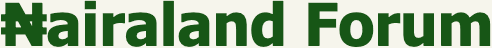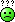#Welcome, Guest: Register On Nairaland / LOGIN! / Trending / Recent / New
Stats: 2,934,515 members, 7,099,358 topics. Date: Monday, 03 October 2022 at 05:44 AM

## Why Dividing Any Number By Zero Is Mathematically Undefined? - Education - Nairaland

(1) (Reply) (Go Down)

 Why Dividing Any Number By Zero Is Mathematically Undefined? by flemsy15(m): 7:30pm On Jan 14, 2021 In sequence to the initial thread where someone asked "why the answer of multiplying any number by zero always gives zero as the answer. I've always wanted to ask this question, maybe the mathematicians in the house can give me a clearer answer.Why is it that when you divide 0(zero) by any number, it gives zero as the answer; but when you divide a number by zero , the answer is undefined, gives error or as some would say, "it's mathematically impossible"FP please. Re: Why Dividing Any Number By Zero Is Mathematically Undefined? by Nobody: 8:39pm On Jan 14, 2021 flemsy15:In sequence to the initial thread where someone asked "why the answer of multiplying any number by zero always gives zero as the answer. I've always wanted to ask this question, maybe the mathematicians in the house can give me a clearer answer.Why is it that when you divide 0(zero) by any number, it gives zero as the answer; but when you divide a number by zero , the answer is undefined, gives error or as some would say, "it's mathematically impossible"FP please.Division by zero means division by nothing which is meaningless or undefined. 2 Likes Re: Why Dividing Any Number By Zero Is Mathematically Undefined? by jpawjpaw(m): 12:21pm On Jan 15, 2021 In programming it is called fatal error!!You can't divide a number by nothing . Re: Why Dividing Any Number By Zero Is Mathematically Undefined? by coronavirus19: 2:42pm On Jan 15, 2021 flemsy15:In sequence to the initial thread where someone asked "why the answer of multiplying any number by zero always gives zero as the answer. I've always wanted to ask this question, maybe the mathematicians in the house can give me a clearer answer.Why is it that when you divide 0(zero) by any number, it gives zero as the answer; but when you divide a number by zero , the answer is undefined, gives error or as some would say, "it's mathematically impossible"FP please.Alright Op to answer your question I would prove here that 1=2 following all mathematical rules except that a number can’t be divided by zero.1=2Let a=b - - - - - - - 1Multiply both sides by aa^2 = ab - - - - - - - - 2Subtract b^2 from both sidesa^2 - b^2 = ab - b^2 - - - - - - - - - 3Diff of two squares in L.S(a-b)(a+b) = b(a-b) - - - - - - - - - - 4Divide B.S by (a-b) - - - - - - - - - 5(a+b) = b - - - - - - - - - 6Since a = bb + b = b2b = b2 = 1The problem here is in step 5 because a = b meaning a - b = 0 which should automatically cut short that proof because by dividing by zero, their is a whole lot of shit you can get out of it. That is why it is always undefined. 3 Likes